D. Energetics

Before redshift measurements were available the GRB energy was estimated from the BATSE catalogue by fitting an (isotropic) luminosity function to the flux distribution (see e.g Cohen and Piran , Guetta et al. , Loredo and Wasserman , Schmidt [379, 380, 381] and many others). This lead to a statistical estimate of the luminosity function of a distribution of bursts.

These estimates were revolutionized with the direct determination of the redshift for individual bursts. Now the energy could be estimated directly for specific bursts. Given an observed-ray fluence and the redshift to a burst one can easily estimate the energy emitted in-rays, E, iso assuming that the emission is isotropic (see Bloom et al.  for a detailed study including k corrections). The inferred energy, E, iso was the isotropic energy, namely the energy assuming that the GRB emission is isotropic in all directions. The energy of the first burst with a determined redshift, GRB 970508, was around 1051 ergs. However, as afterglow observations proceeded, alarmingly large values (e.g. 3.4 × 1054ergs for GRB 990123) were measured for E, iso. The variance was around three orders of magnitude.

However, it turned out [344, 374] that GRBs are beamed and E, iso would not then be a good estimate for the total energy emitted in-rays. Instead: E(2 / 2) E, iso. The angle,, is the effective angle of-ray emission. It can be estimated from tb, the time of the break in the afterglow light curve :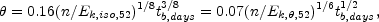(4)

where tb, days is the break time in days. Ek, iso, 52 is "isotropic equivalent" kinetic energy, discussed below, in units of 1052 ergs, while Ek,, 52 is the real kinetic energy in the jet i.e: Ek,, 52 = (2 / 2)Ek, iso, 52. One has to be careful which of the two energies one discusses. In the following I will usually consider, unless specifically mentioned differently, Ek, iso, 52, which is also related to the energy per unit solid angle as: Ek, iso, 52 / 4. The jet break is observed both in the optical and in the radio frequencies. Note that the the observational signature in the radio differs from that at optical and X-ray [159, 374] (see Fig. 25) and this provides an additional confirmation for this interpretation.

Frail et al.  estimated Efor 18 bursts, finding typical values around 1051 ergs (see also Panaitescu and Kumar ). Bloom et al.  find E= 1.33 × 1051 h65-2 erg and a burst-to-burst variance about this value ~ 0.35 dex, a factor of 2.2. This is three orders of magnitude smaller than the variance in the isotropic equivalent E. A compilation of the beamed energies from , is shown in Figs 12 and 13. It demonstrates nicely this phenomenon. The constancy of Eis remarkable, as it involves a product of a factor inferred from the GRB observation (the-rays flux) with a factor inferred from the afterglow observations (the jet opening angle). However, Emight not be a good estimate for Etot, the total energy emitted by the central engine. First, an unknown conversion efficiency of energy to-rays has to be considered: Etot =-1 E=-1(2 / 2) E, iso. Second, the large Lorentz factor during the-ray emission phase, makes the observed Erather sensitive to angular inhomogeneities of the relativistic ejecta . The recent early observations of the afterglow of GRB 021004 indicate that indeed a significant angular variability of this kind exists [271, 274].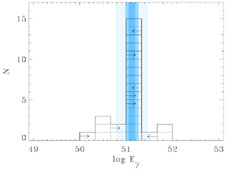Figure 12. A histogram of GRB energies (E) with three equal logarithmic spacings per decade. The histogram shows a narrow distribution of GRB energies about the standard energy E= 1.33 . 1051 ergs , with an error of= 0.07dex. The observed burst-to-burst rms spread is 0.35dex (a factor of 2.23) about this value. Bands of 1, 2, and 5about the standard energy are shown. There are five identifiable outliers, which lie more than 5from the mean, however, there is currently no basis other than discrepant energy to exclude these bursts from the sample (.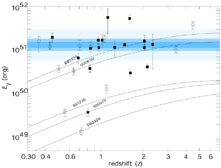Figure 13. GRB energy release versus redshift. Bands of 1, 2, and 5about the mean energy E= 1.33 . 1051 ergs are shown. Plotted are the trajectories of five GRBs with no known spectroscopic redshift (labeled with star symbols). While the energies of GRB 980329 and GRB 000630 could be consistent with the standard value at redshifts beyond z ~ 1.5, at no redshift could the energies of GRB 980326 and GRB 980519 be consistent (Bloom et al. .

The kinetic energy of the flow during the adiabatic afterglow phase, Ek is yet another energy measure that arises. This energy (per unit solid angle) can be estimated from the afterglow light curve and spectra. Specifically it is rather closely related to the observed afterglow X-ray flux [109, 201, 310]. As this energy is measured when the Lorentz factor is smaller it is less sensitive than Eto angular variability. The constancy of the X-ray flux  suggest that this energy is also constant. Estimates of Ek,show that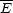3k,, namely the observed "beamed" GRB energy is larger than the estimated "beamed" kinetic energy of the afterglow. Frail et al. , however, find thatk,, namely that the two energies are comparable.

An alternative interpretation to the observed breaks is that we are viewing a "universal" angle dependent, namely, "structured" jet - from different viewing angles [219, 347, 446]. The observed break corresponds in this model to the observing angleand not to the opening angle of the jet. This interpretation means that the GRB beams are wide and hence the rate of GRBs is smaller than the rate implied by the usual beaming factor. On the other hand it implies that GRBs are more energetic. Guetta et al.  estimate that this factor (the ratio of the fixed energy of a "structured" jet relative to the energy of a uniform jet to be ~ 7. However they find that the observing angle distribution is somewhat inconsistent with the simple geometric one that should arise in universal structured jets (see also Nakar et al. , Perna et al. ). The energy-angle relation discussed earlier require (see Section VIII below) an angle dependent jet with E()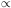-2.

Regardless of the nature of the jet (universal structured jet or uniform with a opening angle that differs from one burst to another) at late time it becomes non relativistic and spherical. With no relativistic beaming every observer detects emission from the whole shell. Radio observations at this stage enable us to obtain a direct calorimetric estimate of the total kinetic energy of the ejecta at late times  Estimates performed in several cases yield a comparable value for the total energy.

If GRBs are beamed we should expect orphan afterglows (see Section VIIK): events in which we will miss the GRB but we will observe the late afterglow that is not so beamed. A comparison of the rate of orphan afterglows to GRBs will give us a direct estimate of the beaming of GRBs (and hence of their energy). Unfortunately there are not even good upper limits on the rate of orphan afterglows. Veerswijk  consider the observations within the Faint Sky Variability Survey (FSVS) carried out on the Wide Field Camerea on teh 2.5-m Isacc Newton Telescope on La Palma. This survey mapped 23 suare degree down to a limiting magnitude of about V = 24. They have found one object which faded and was not detected after a year. However, its colors suggest that it was a supernova and not a GRB. Similarly, Vanden Berk et al.  find a single candidate within the Sloan Digital Sky Survey. Here the colors were compatible with an afterglow. However, later it was revealed that this was a variable AGN and not an orphan afterglow. As I discuss later this limits are still far to constrain the current beaming estimates (see Section VIIK).

One exception is for late radio emission for which there are some limits [215, 298]. Levinson et al.  show that the number of orphan radio afterglows associated with GRBs that should be detected by a flux-limited radio survey is smaller for a smaller jet opening angle. This might seen at first sight contrary to expectation as narrower beams imply more GRBs. But, on the other hand, with narrower beams each GRB has a lower energy and hence its radio afterglow is more difficult to detect. Overall the second factor wins. Using the results of FIRST and NVSS surveys they find nine afterglow candidates. If all candidates are associated with GRBs then there is a lower limit on the beaming factor of f-1b(2 / 2) > 13. If none are associated with GRBs they find f-1b > 90. This give immediately a corresponding upper limit on the average energies of GRBs. Guetta et al.  revise this values in view of a recent estimates of the correction to the rate of GRBs to: f-1b = 40.

When considering the energy of GRBs one has to remember the possibility, as some models suggest, that an additional energy is emitted which is not involved in the GRB itself or in the afterglow. van Putten and Levinson , for example, suggest that a powerful Newtonian wind collimates the less powerful relativistic one. The "standard jet" model also suggests a large amount of energy emitted sideways with a lower energy per solid angle and a lower Lorentz factors. It is interesting to note that the calorimetric estimates mentioned earlier limit the total amount of energy ejected regardless of the nature of the flow. More generally, typically during the afterglow matter moving with a lower Lorentz factor emits lower frequencies. Hence by comparing the relative beaming of afterglow emission in different wavelength one can estimate the relative beaming factors, f-1b(E), at different wavelength and hence at different energies. Nakar and Piran  use various X-ray searches for orphan X-ray afterglow to limit the (hard) X-ray energy to be at most comparable to the-rays energy. This implies that the total energy of matter moving at a Lorentz factor of ~ 40 is at most comparable to the energy of matter moving with a Lorentz factor of a few hundred and producing the GRB itself. At present limits on optical orphan afterglow are insufficient to set significant limits on matter moving at slower rate, while as mentioned earlier radio observations already limit the overall energy output.

These observations won't limit, of course, the energy emitted in gravitational radiation, neutrinos, Cosmic Rays or very high energy photons that may be emitted simultaneously by the source and influence the source'e energy budget without influencing the afterglow.